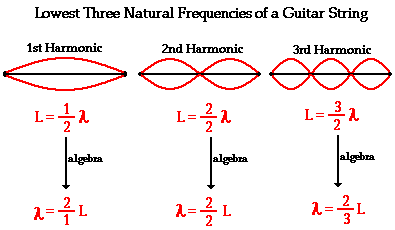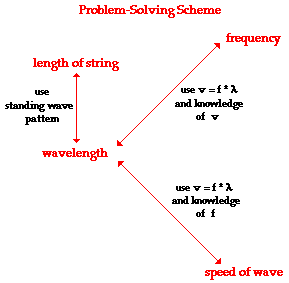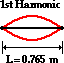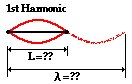Sound Waves and Music - Lesson 5 - Physics of Musical Instruments

# Guitar Strings

A guitar string has a number of frequencies at which it will naturally vibrate. These natural frequencies are known as the harmonics of the guitar string. As mentioned earlier, the natural frequency at which an object vibrates at depends upon the tension of the string, the linear density of the string and the length of the string. Each of these natural frequencies or harmonics is associated with a standing wave pattern. The specifics of the patterns and their formation were discussed in Lesson 4. For now, we will merely summarize the results of that discussion. The graphic below depicts the standing wave patterns for the lowest three harmonics or frequencies of a guitar string.### Problem-Solving Scheme

The wavelength of the standing wave for any given harmonic is related to the length of the string (and vice versa). If the length of a guitar string is known, the wavelength associated with each of the harmonic frequencies can be found. Thus, the length-wavelength relationships and the wave equation (speed = frequency * wavelength) can be combined to perform calculations predicting the length of string required to produce a given natural frequency. And conversely, calculations can be performed to predict the natural frequencies produced by a known length of string. Each of these calculations requires knowledge of the speed of a wave in a string. The graphic below depicts the relationships between the key variables in such calculations. These relationships will be used to assist in the solution to problems involving standing waves in musical instruments.To demonstrate the use of the above problem-solving scheme, consider the following problem and its detailed solution.

### Example Problem #1

The speed of waves in a particular guitar string is 425 m/s. Determine the fundamental frequency (1st harmonic) of the string if its length is 76.5 cm.

The solution to the problem begins by first identifying known information, listing the desired quantity, and constructing a diagram of the situation.

 Given: v = 425 m/s L = 76.5 cm = 0.765 m Find: f1 = ?? Diagram:The problem statement asks us to determine the frequency (f) value. From the graphic above, the only means of finding the frequency is to use the wave equation (speed=frequency • wavelength) and knowledge of the speed and wavelength. The speed is given, but wavelength is not known. If the wavelength could be found, then the frequency could be easily calculated. In this problem (and any problem), knowledge of the length and the harmonic number allows one to determine the wavelength of the wave. For the first harmonic, the wavelength is twice the length. This relationship is derived from the diagram of the standing wave pattern (and was explained in detail in Lesson 4). This relationship, which works only for the first harmonic of a guitar string, is used to calculate the wavelength for this standing wave.

Wavelength = 2 • Length

Wavelength = 2 • 0.765 m

Wavelength = 1.53 m

Now that wavelength is known, it can be combined with the given value of the speed to calculate the frequency of the first harmonic for this given string. This calculation is shown below.

speed = frequency • wavelength

frequency = speed / wavelength

frequency = (425 m/s) / (1.53 m)

frequency = 278 Hz

Most problems can be solved in a similar manner. It is always wise to take the extra time needed to set the problem up; take the time to write down the given information and the requested information and to draw a meaningful diagram. These preparatory steps become more important as problems become more difficult.

Seldom in physics are two problems identical. The tendency to treat every problem the same way is perhaps one of the quickest paths to failure. It is important to combine good problem-solving skills (part of which involves the discipline to set the problem up) with a solid grasp of the relationships among variables. Avoid the tendency to memorize approaches to different types of problems. To further your understanding of these relationships and the use of the above problem-solving scheme, examine the following problem and its solution.

### Example Problem #2

Determine the length of guitar string required to produce a fundamental frequency (1st harmonic) of 256 Hz. The speed of waves in a particular guitar string is known to be 405 m/s.

The solution to the problem begins by first identifying known information, listing the desired quantity, and constructing a diagram of the situation.

 Given: v = 405 m/s f1 = 256 Hz Find: L = ?? Diagram:The problem statement asks us to determine the length of the guitar string. From the graphic above, the only means of finding the length of the string is from knowledge of the wavelength. But the wavelength is not known. However, the frequency and speed are given, so one can use the wave equation (speed = frequency • wavelength) and knowledge of the speed and frequency to determine the wavelength. This calculation is shown below.

speed = frequency • wavelength

wavelength = speed / frequency

wavelength = (405 m/s) / (256 Hz)

wavelength = 1.58 m

Now that the wavelength is found, the length of the guitar string can be calculated. For the first harmonic, the length is one-half the wavelength. This relationship is derived from the diagram of the standing wave pattern (and was explained in detail in Lesson 4). It may also be evident to you by looking at the standing wave diagram drawn above. This relationship between wavelength and length, which works only for the first harmonic of a guitar string, is used to calculate the wavelength for this standing wave pattern.

Length = (1/2) • Wavelength

Length = (1/2) • Wavelength

Length = 0.791 m

If you have successfully followed the logic in the above two example problems, take a try at the following practice problems. As you proceed, be sure to be mindful of the numerical relationships involved in such problems. And if necessary, refer to the graphic above.

### We Would Like to Suggest ...Why just read about it and when you could be interacting with it? Interact - that's exactly what you do when you use one of The Physics Classroom's Interactives. We would like to suggest that you combine the reading of this page with the use of our Standing Wave Patterns Interactive. You can find it in the Physics Interactives section of our website. The Standing Wave Patterns Interactive provides the learner an environment for exploring the formation of standing waves, standing wave patterns, and mathematical relationships for standing wave patterns.

1. A guitar string with a length of 80.0 cm is plucked. The speed of a wave in the string is 400 m/sec. Calculate the frequency of the first, second, and third harmonics.

2. A pitch of Middle D (first harmonic = 294 Hz) is sounded out by a vibrating guitar string. The length of the string is 70.0 cm. Calculate the speed of the standing wave in the guitar string.

3. A frequency of the first harmonic is 587 Hz (pitch of D5) is sounded out by a vibrating guitar string. The speed of the wave is 600 m/sec. Find the length of the string.

Next Section: